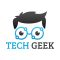Home » Core Java » Declare Array Java ExampleThe author is graduated in Electronics & Telecommunication. During his studies, he has been involved with a significant number of projects ranging from programming and software engineering to telecommunications analysis. He works as a technical lead in the information technology sector where he is primarily involved with projects based on Java/J2EE technologies platform and novel UI technologies.

# Declare Array Java Example

Hello readers, in this tutorial, we will learn how to declare ARRAYS in Java programming language.

You can watch the following video and learn how to use arrays in Java:

## 1. Introduction

Java programming offer Arrays to store the elements of a similar data-type. Arrays,

• Once defined, the array size is fixed and cannot increase to accommodate more elements
• The first element in an array starts with index `0` and so on
• Elements share a common memory location

### 1.1 Types of Array

Java programming language supports two types of arrays –

• Single dimensional array
• Multi-dimensional or 2D array – This array is rarely used in a programming language, so we are listing here for knowledge purposes only

### 1.2 How to use an Array?

In Java programming language, using an array is 3-step process i.e.

1. Declaring an Array
2. Constructing an Array
3. Initializing an Array

Let us see a few examples to understand the process of using an array. To start with, we are hoping that users at present have their preferred Ide installed on their machines. For easy usage, I am using Eclipse Ide on a Windows operating system.

## 2. Declare Array Java Example

In this example, we’ll demonstrate three different examples to illustrate the use of Arrays in the Java programming language. For a better understanding, developers can execute the below code in Eclipse Ide.

### 2.1 First Array Example

Let us see the simple example of Java Array, where we’ll declare, instantiate, initialize, and traverse an array.

Firstarrayexample.java

```package com.java.arrays;

// Java program to illustrate declaration, instantiation, initialization, and traversing the array.
public class Firstarrayexample {

public static void main(String[] args) {

// Array declaration and instantiation.
// Allocating memory for 7 integers.
int[] arr = new int;

// Array initialization.
for(int count=0; count<7; count++) {
arr[count] = count+1;
}

// Calculating the array length.
int arrayLength = arr.length;
// Traversing the array.
for(int i=0; i<arrayLength; i++) {
System.out.println("Element at index " + i +" : "+ arr[i]);
}
}
}
```

If everything goes well, the following output will be printed in the Ide console.

Output

```Element at index 0 : 1
Element at index 1 : 2
Element at index 2 : 3
Element at index 3 : 4
Element at index 4 : 5
Element at index 5 : 6
Element at index 6 : 7
```

### 2.2 Second Array Example

Let us see an example, where we’ll declare, instantiate, initialize, and traverse a multi-dimensional array.

Secondarrayexample.java

```package com.java.arrays;

// Java program to illustrate the use of a multidimensional array.
public class Secondarrayexample {

public static void main(String[] args) {
// Declaring and initializing the 2D array.
int arr[][] = {{1,2,3}, {4,5,6}, {7,8,9}};

// Printing the 2D array.
for(int i=0; i<3; i++) {
for(int j=0; j<3; j++) {
System.out.print(arr[i][j] + " ");
}
System.out.println();
}
}
}
```

If everything goes well, the following output will be printed in the Ide console.

Output

```1 2 3
4 5 6
7 8 9
```

### 2.3 Array of Objects Example

Let us see an example, where we’ll declare, instantiate, initialize, and traverse an array of objects just like an array of data-type in Java.

Thirdarrayexample.java

```package com.java.arrays;

class Employee {
public int id;
public String name;

Employee() { }

Employee(final int id, final String name) {
this.id = id;
this.name = name;
}

@Override
public String toString() {
return "Employee [id=" + id + ", name=" + name + "]";
}
}

// Java program to illustrate the use of an array of objects.
public class Thirdarrayexample {

public static void main(String[] args) {
Employee[] emp_array = new Employee;
emp_array = new Employee(101, "Abc");
emp_array = new Employee(102, "Pqr");
emp_array = new Employee(103, "Xyz");

for(int x=0; x<emp_array.length; x++) {
System.out.println("Element at " + x + " : " + emp_array[x].toString());
}
}
}
```

If everything goes well, the following output will be printed in the Ide console.

Output

```Element at 0 : Employee [id=101, name=Abc]
Element at 1 : Employee [id=102, name=Pqr]
Element at 2 : Employee [id=103, name=Xyz]
```

That is all for this tutorial and I hope the article served you whatever you were looking for. Happy Learning and do not forget to share!

## 3. Conclusion

In this tutorial, we learned how to declare and instantiate an `ARRAY` in Java. Developers can download the sample application as an Eclipse project in the Downloads section.

This was an example of ARRAYS in Java programming language.

You can download the full source code of this example here: Declare Array Java Example
0 0 vote
Article Rating

# Do you want to know how to develop your skillset to become a Java Rockstar?

## Subscribe to our newsletter to start Rocking right now!

### and many more ....

Subscribe
Notify of